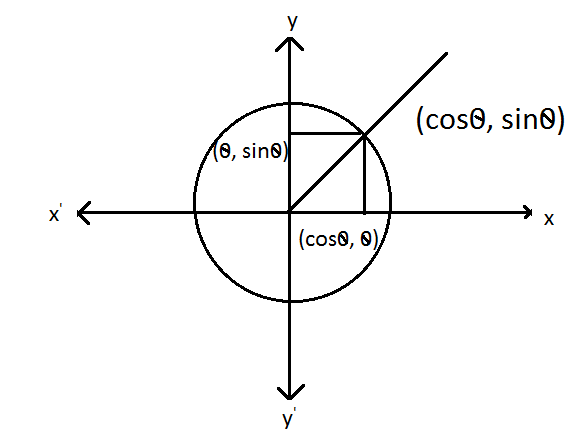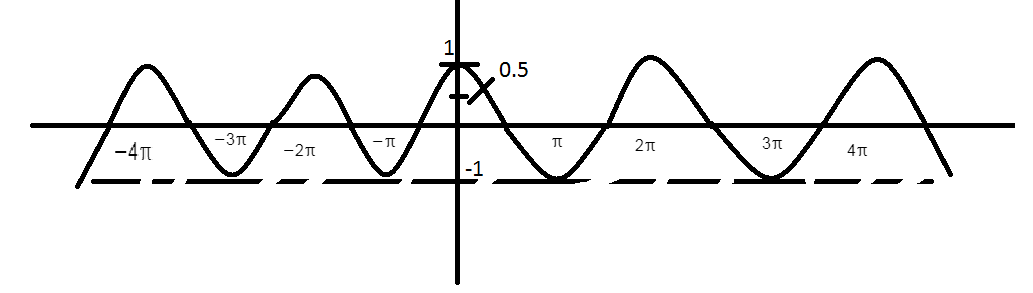QuestionAnswers

# Find the minimum value of $\cos \theta + \cos 2\theta$for all real value of $\theta$Verified
128.1k+ views
Hint: The cosine function is a periodic function which is very important in trigonometry. The simplest way to understand the cosine function is to use the unit circle. For a given angle measure (-) draw a unit circle on the coordinate plane and draw the angle centered at the origin with one side as the positive $x - axiz$ the $x -$ coordinate of the point where the other side of the angle intersects the circle is $\cos (\theta )$, and the y coordinate is $\sin (\theta )$The amplitude of a wave is basically a measure of its height. Because that height is constantly changing amplitude can be different form moment to moment. If the wave has a regular up and down shape, like a cosine, sine wave. The amplitude is defined as the fact act distance the wave gets from its center.Which shows that
Max value of $\cos \,\,\,\,\,\, + 1$
Min value of $\cos \,\,\,\,\,\, - 1$

Given function is
$= \cos 2\theta + \cos \theta$
$= 2{\cos ^2}\theta - 1 + \cos \theta$ $\left[ {\cos 2\theta - 2{{\cos }^2}\theta - 1} \right]$
$= {\cos ^2}\theta - \dfrac{1}{2}\cos \theta - \dfrac{1}{2}$$\left[ {dividing\;by\,2} \right]$
$= ({\cos ^2}\theta + \dfrac{1}{2}\cos \theta + \dfrac{1}{{16}}) - \dfrac{1}{{16}} - \dfrac{1}{2}$ $\left[ {For\,factorize\,add\& substract\,\dfrac{1}{{16}}} \right]$
$= {(\cos \theta + \dfrac{1}{2})^2} - (\dfrac{{1 + 8}}{{16}})$ $\left[ {Making\,the\,whole\,square} \right]$
$= {(\cos \theta + \dfrac{1}{4})^2} - (\dfrac{9}{{16}})$
Now for minimum value if $\cos \theta = 0$
i.e.$= \dfrac{{ - 9}}{{16}}$
Required minimum value of $\cos \theta + \cos 2\theta$ for all real values of $\theta$ is $= \dfrac{{ - 9}}{{16}}$
$(\cos + \dfrac{1}{2})\dfrac{1}{2}O$
$\cos \theta = \dfrac{1}{2}$

Note: If we take negative value of $\cos \theta$ because it’s a square so at last it become positive.
Minimum value of $\cos \theta$ is -1 when $\theta = {180^0}$ Maximum value $\cos \theta$ 1 when$\theta$ is $O,360$ Min-Max table.